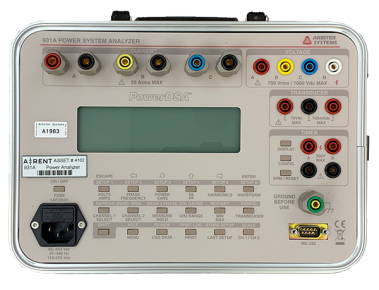# Arbiter 931A

Power System Analyzer

Details

• With PowerDSA Digital Signal Analysis, measures all of the basic quantities: true-rms voltage and current, frequency and phase angle, as well as power quantities such as watts (W), watthours (Wh), volt-amperes (VA), volt-ampere hours (VAh), volt-amperes reactive (VAR), volt-amperes reactive hours (VARh), and power factor (PF), all with 0.11 % basic accuracy
• Display view options include basic three-phase, vector, voltage/current sequence and energy
• Power trend monitor and recording system to verify phase relation, phase rotation, power direction, load balance and more
• Measures CT/PT ratios